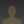0%
0%

# Polyline Offset

Writing C code to offset a polyline

Similar projects worth following
193 views
0 followers
It not as simple as it first appears!

## Scope

The scope of this project is to document the coding of a rather complex problem.

## Basic Code

To begin I need:

• a "segment" intersection routine
• an offset routine
• some test data

I have decided to truncate intersection "rays" in the basic code.

Note: a segment is line bounded by two points while a ray is an infinitely long line.

## Resolving Cross-Overs

Resolving cross-overs can wait until the basic code works  as well as it can.

## Initial Experiments

Initially to test the two main routines I built a program that steps through increasing angles for two joined segments:

Here is the first case where the offset segments intersect:

Here is the third case where the intersection exceeds the extended offset segment. In this case I have truncated the offset polyline:

This truncated offset polyline is fine for CNC mill or laser cutting applications.

The last case is problematic:

I have to either accept this or a ray intersection.

After a lot experimentation, I decided to accept the ray intersection.

A final case to consider is co-linear segments. The two spikes are co-linear segments:

## Next Set of Experiments

Here is my test data (the white closed polyline) for the next set of experiments:

For this experiment I filtered the original (i.e. the white polyine) for near co-linear segments and very short segments. Although the original polyline appears symmetrical, the location of the points are not.

Hers is the contraction case:

If I expand or contract the original polyline sufficiently problems appear:

Note the offset polyline cross-over.

And:

In this case the offset polyline has reversed direction. The offset polyline is also distorted due to the order of the offset point intersection truncation.

## Status

At this point of time I have basic working code. There is potential to improve the code by resolving cross-overs and taking another approach to ray truncation.

AlanX

### PolyLineOffset.run

- 30.56 kB - 06/17/2019 at 12:57

### libezx.a

x-archive - 28.44 kB - 06/16/2019 at 09:14

### ezx_graphics.pdf

Adobe Portable Document Format - 92.72 kB - 06/16/2019 at 09:14

### ezxdisp.h

x-chdr - 4.33 kB - 06/16/2019 at 09:14

### PloyLineOffset.c

text/x-csrc - 7.74 kB - 06/16/2019 at 09:13

## Cross-Over Removal

Added code to remove co-linear points (spikes) and cross-overs. While cross-over removal is rather ambiguous (i.e. which is the cross-over and which is the polyline?). Rather than get too "deep", I just decided to limit the search to only half of the polyline (by point count). Here is the expansion case, before:

and after:

Here is the contractions case, before:

and after:

Well, that just about does it.

AlanX

## Basic Code

The basic code is not too bad.

Here is my segment intersection routine:

```int intersect(
double px0,double py0,double px1,double py1,
double px2,double py2,double px3,double py3,
double *px,double *py)
{
double det,s,t;

// Test if co-linear
det=(px3-px2)*(py0-py1)-(px0-px1)*(py3-py2);
if (fabs(det)>1e-12) {
// Find ray intersection
s=((px1-px0)*(py0-py2)-(py1-py0)*(px0-px2))/det;
t=((px3-px2)*(py0-py2)-(py3-py2)*(px0-px2))/det;
*px=px0+t*(px1-px0);
*py=py0+t*(py1-py0);
if ((s>=0.0)&&(s<=1.0)&&(t>=0.0)&&(t<=1.0)) {
// Segment Intersection
return 1;
} else {
// Ray Intersection
return 2;
}
} else {
// In my special case (for a polyline), I want the last point.
*px=px3;
*py=py3;
return 0;
}
}```

The offset routine, considers the cases returned from the intersection routine:

```int makeOffset(
double x1,double y1,double xc,double yc,double x2,double y2,double Offset,
double *xi1,double *yi1,double *xi2,double *yi2)
{
double dx1,dy1,dl1,xo1,yo1,xc1,yc1;
double dx2,dy2,dl2,xo2,yo2,xc2,yc2;
double xi,yi;
int Test;

// Segment lengths
dx1=xc-x1;
dy1=yc-y1;
dl1=sqrt(dx1*dx1+dy1*dy1);
dx2=xc-x2;
dy2=yc-y2;
dl2=sqrt(dx2*dx2+dy2*dy2);
if ((dl1>1e-12)&&(dl2>=1e-12)) {
dx1=dx1/dl1*Offset;
dy1=dy1/dl1*Offset;
dx2=dx2/dl2*Offset;
dy2=dy2/dl2*Offset;
xo1=x1-dy1;
yo1=y1+dx1;
if (Offset>=0.0) {
xc1=xc-dy1+dx1;
yc1=yc+dx1+dy1;
xc2=xc+dy2+dx2;
yc2=yc-dx2+dy2;
} else {
xc1=xc-dy1-dx1;
yc1=yc+dx1-dy1;
xc2=xc+dy2-dx2;
yc2=yc-dx2-dy2;
}
xo2=x2+dy2;
yo2=y2-dx2;
Test=intersect(xo1,yo1,xc1,yc1,xc2,yc2,xo2,yo2,&xi,&yi);
if (Test==1) {
// Segment Plus Offset Intercept
*xi1=xi;
*yi1=yi;
*xi2=xi;
*yi2=yi;
return 1;
} else if (Test==2) {
double area=((y1-y2)*(xc-x2)-(yc-y2)*(x1-x2));
if (((area<0)&&(Offset<0))||((area>0)&&(Offset>0))) {
// External Ray Intercept (use truncated offsets)
*xi1=xc1;
*yi1=yc1;
*xi2=xc2;
*yi2=yc2;
return 2;
} else {
// Internal Ray Intercept (use intercept)
*xi1=xi;
*yi1=yi;
*xi2=xi;
*yi2=yi;
return 3;
}
} else {
// Co-linear Intercept
*xi1=xi;
*yi1=yi;
*xi2=xi;
*yi2=yi;
return 4;
}
}
return 0;
}

```

One problem with the offset code is what to do with very short segments ?

My solution filter the polyline for:

• segments (points) that are nearly co-linear,
• segments that are very short.
```  // Filter Points (Open Polyline)
Test=true;
while (Test) {
Test=false;
int count=0;
for (i=0;i<=N;i++) {
count++;
if (count>=3) {
double area=fabs((Y[i-1]-Y[i-2])*(X[i]-X[i-2])-(Y[i]-Y[i-2])*(X[i-1]-X[i-2]));
double length=sqrt((Y[i]-Y[i-2])*(Y[i]-Y[i-2])+(X[i]-X[i-2])*(X[i]-X[i-2]));
if ((length*fabs(Offset)>area*100)||(fabs(Offset)>length*10)) {
D[i-1]=true;
Test=true;
count=1;
}
}
}
int j=0;
for (i=0;i<=N;i++) {
if (!D[i]) {
X[j]=X[i];
Y[j]=Y[i];
D[j]=false;
j++;
}
}
N=j-1;
}
```

Finally the make Polyline Offset code:

```  // Polyline Offset
x1=X[N-2];
y1=Y[N-2];
xc=X[N-1];
yc=Y[N-1];
x2=X;
y2=Y;
makeOffset(x1,y1,xc,yc,x2,y2,Offset,&xc1,&yc1,&xc0,&yc0);
NT=0;
XT[NT]=xc0;
YT[NT]=yc0;
NT++;
for (i=0;i<N;i++) {
i1=(i+N-1)%N;
ic=(i+N)%N;
i2=(i+N+1)%N;
x1=X[i1];
y1=Y[i1];
xc=X[ic];
yc=Y[ic];
x2=X[i2];
y2=Y[i2];

ezx_line_2d(disp,width/2+(int)(scale*x1),height/2-(int)(scale*y1),width/2+(int)(scale*xc),height/2-(int)(scale*yc),&ezx_white,1);
Test=makeOffset(x1,y1,xc,yc,x2,y2,Offset,&xc1,&yc1,&xc2,&yc2);
if (Test==1) {
// Normal Intercept
ezx_line_2d(disp,width/2+(int)(scale*xc0),height/2-(int)(scale*yc0),width/2+(int)(scale*xc2),height/2-(int)(scale*yc2),&ezx_red,1);
XT[NT]=xc2;
YT[NT]=yc1;
NT++;
} else if (Test==2) {
// Truncated Intercept
ezx_line_2d(disp,width/2+(int)(scale*xc0),height/2-(int)(scale*yc0),width/2+(int)(scale*xc1),height/2-(int)(scale*yc1),&ezx_red,1);
ezx_line_2d(disp,width/2+(int)(scale*xc1),height/2-(int)(scale*yc1),width/2+(int)(scale*xc2),height/2-(int)(scale*yc2),&ezx_red,1);
XT[NT]=xc1;
YT[NT]=yc1;
NT++;
XT[NT]=xc2;
YT[NT]=yc2;
NT++;
} else if (Test==3) {
// Ray Intercept
ezx_line_2d(disp,width/...```

Share

## Similar Projects

Project Owner Contributortsmspace

Project Owner Contributor

### Nand2Tetris in Verilog Part3 - Verilator and SDL2Aria

# Does this project spark your interest?

Become a member to follow this project and never miss any updates